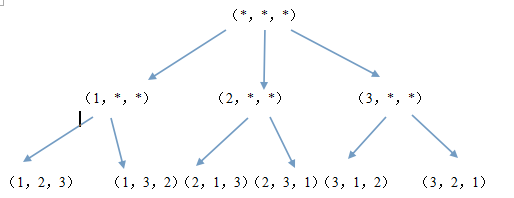###### 闲敲代码、落灯花
What's past is prologue

# 算法--生成1~n的排列

（1，2，3），（1，3，2），（2，1，3），（2，3，1），（3，1，2），（3，2，1）。``````#include<cstdio>
#include <cstdlib>
using namespace std;

void permutation(int *a,int n,int cur){
if(cur==n){
for(int i=0;i<n;i++)printf("%d ",a[i]);printf("\n");
}
else {
for(int j=1;j<=n;j++){
int ok=1;
for(int k=0;k<cur;k++){
if(a[k]==j){
ok=0;break;
}
}
if(ok){
a[cur]=j;
permutation(a,n,cur+1);
}
}
}
}
int main(){
int n;
scanf("%d",&n);
int *a=new int[n];
permutation(a,n,0);
}``````